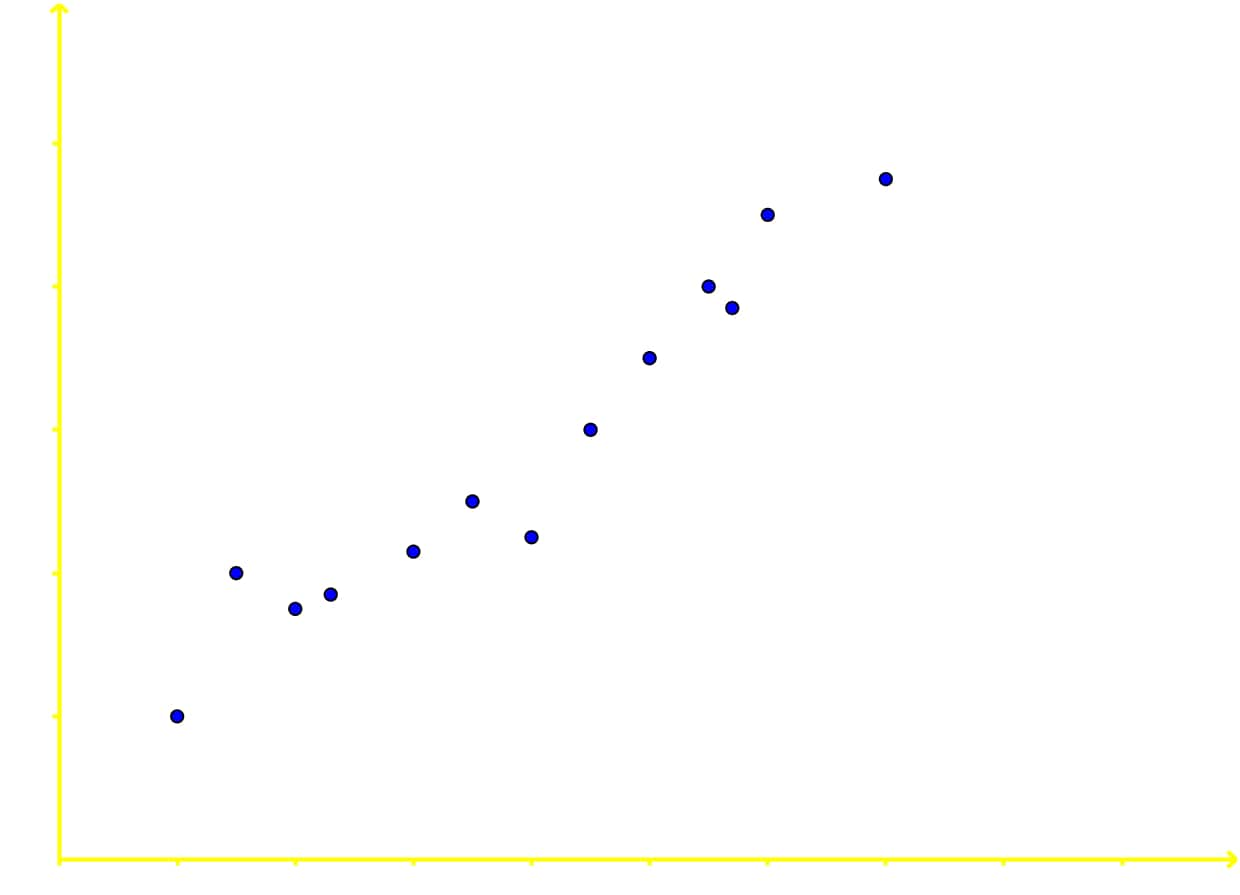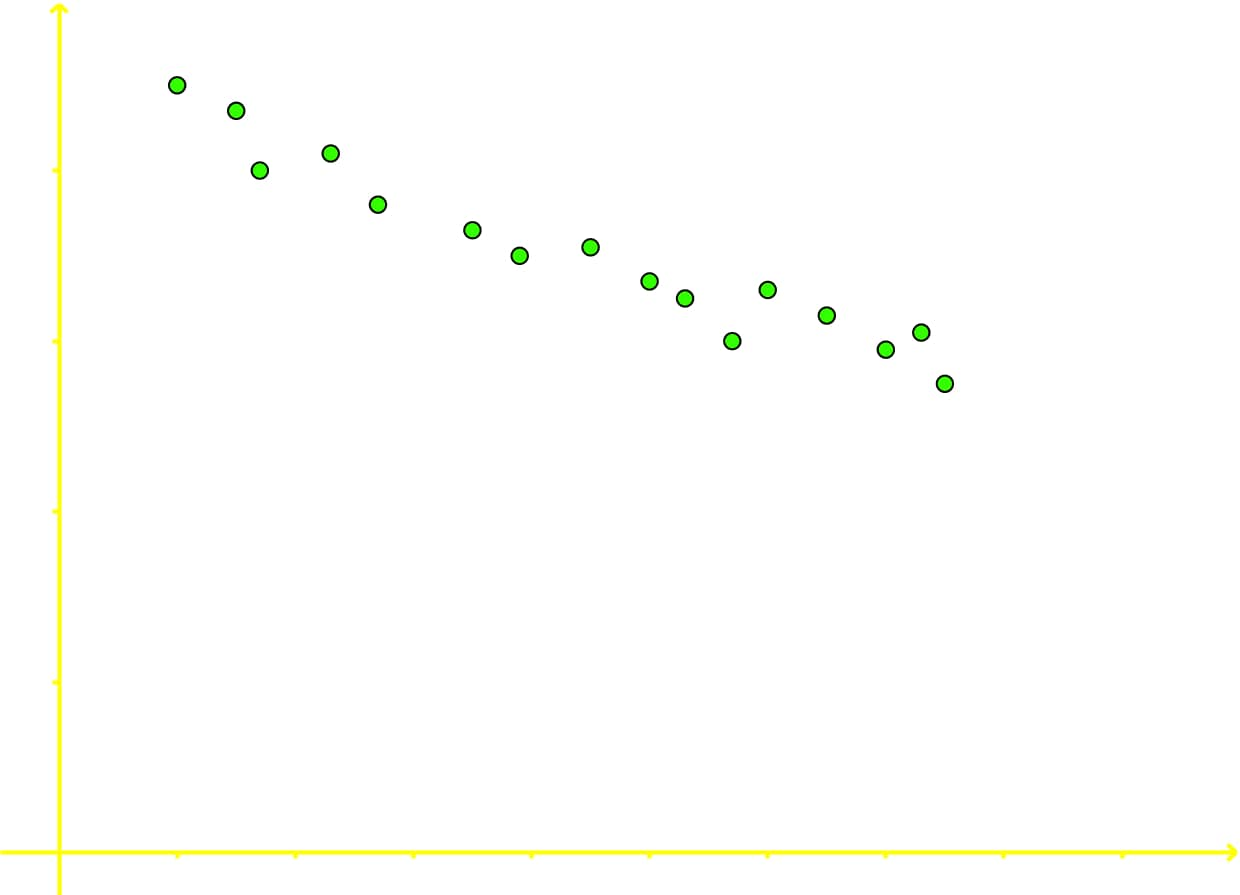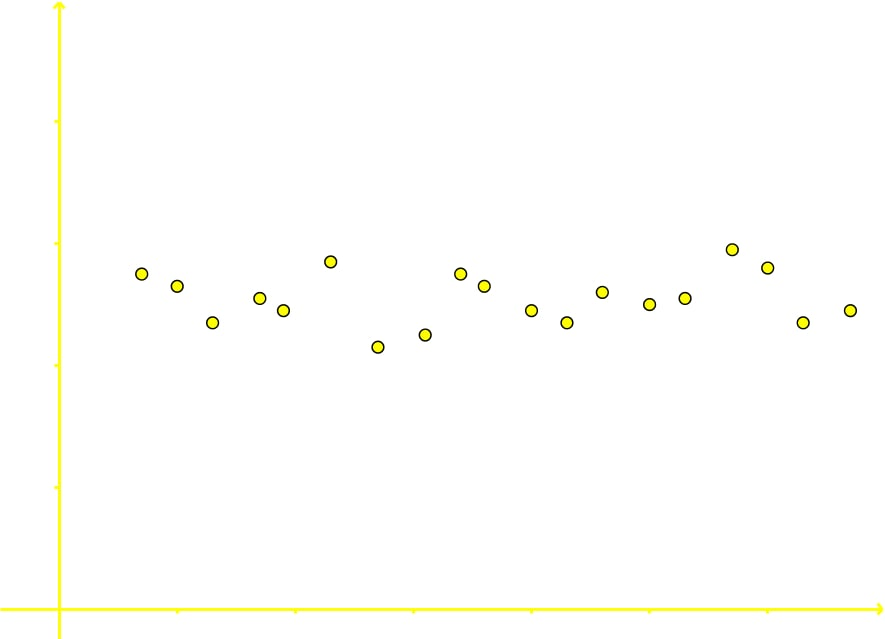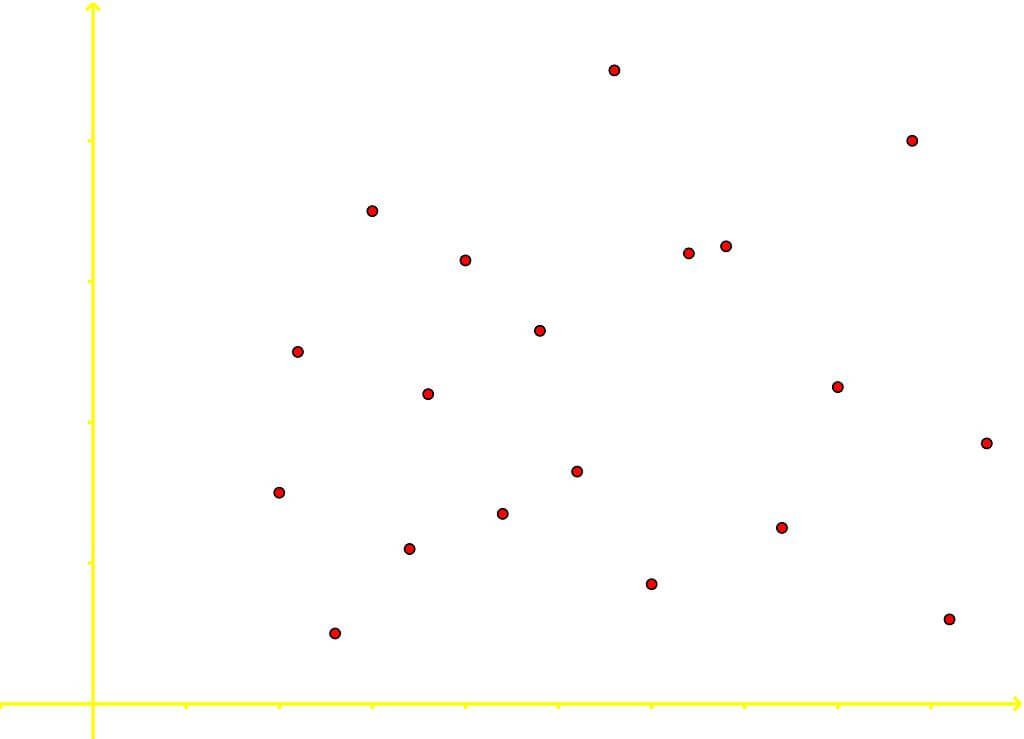# Bivariate, scatter plots and correlation

### Bivariate, scatter plots and correlation

#### Lessons

• Bivariate data is data that has two variables
• Scatter Plots are a graphical representation of two different data sets
• Introduction
What is Bivariate data, and how do we model it?

• 1.
Determining Correlation
For each of the following scatter plots determine whether the bivariate data is positively correlated, negatively correlated, or has no correlation.
a)b)c)d)• 2.
State whether each of the following bivariate data will most likely be positively correlated, negatively correlated or have no correlation:
a)
Amount of gas put into a car's gas tank and the distance that car will travel

b)
Amount of cigarettes smoked and your life expectancy

c)
The amount of time you spend watching TV and the price of rice in China